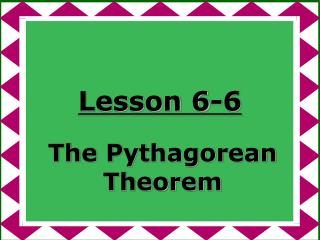DownloadDownload PresentationLesson 6-6

# Lesson 6-6

Télécharger la présentation## Lesson 6-6

- - - - - - - - - - - - - - - - - - - - - - - - - - - E N D - - - - - - - - - - - - - - - - - - - - - - - - - - -
##### Presentation Transcript

1. Lesson 6-6 The Pythagorean Theorem

2. Ohio Content Standards:

3. Ohio Content Standards: Find the square root of perfect squares, and approximate the square root of non-perfect squares.

4. Ohio Content Standards: Find the square root of perfect squares, and approximate the square root of non-perfect squares as consecutive integers between which the root lies; e.g., (square root of 130) is between 11 and 12.

5. Ohio Content Standards: Estimate, compute and solve problems involving scientific notation, square roots and numbers with integer exponents.

6. Ohio Content Standards: Apply order of operations to simplify expressions and perform computations involving integer exponents and radicals.

7. Ohio Content Standards: Estimate the solutions for problem situations involving square and cube roots.

8. Ohio Content Standards: Establish the validity of conjectures about geometric objects, their properties and relationships by counter-example, inductive and deductive reasoning, and critiquing arguments made by others.

9. Ohio Content Standards: Make, test and establish the validity of conjectures about geometric properties and relationships using counterexample, inductive and deductive reasoning, and paragraph or two-column proof, including:a. prove the Pythagorean Theorem;b. prove theorems involving triangle similarity and congruence;c. prove theorems involving properties of lines, angles, triangles and quadrilaterals;d. test a conjecture using basic constructions made with a compass and straightedge or technology.

10. Ohio Content Standards: Use right triangle trigonometric relationships to determine lengths and angle measures.

11. Ohio Content Standards: Apply proportions and right triangle trigonometric ratios to solve problems involving missing lengths and angle measures in similar figures.

12. Pythagorean Theorem

13. Pythagorean Theorem In a right triangle, the square of the lengths of the hypotenuse c is equal to the sum of the squares of the lengths of the legs a and b.

14. Pythagorean Theorem In a right triangle, the square of the lengths of the hypotenuse c is equal to the sum of the squares of the lengths of the legs a and b. a2+ b2 = c2

15. Pythagorean Theorem c a b a2 + b2 = c2

16. Find the length of one leg of a right triangle if the length of the hypotenuse is 4 meters and the length of the other leg is 3 meters. 4 m 3 m

17. If c is the measure of the hypotenuse, find the missing measure. Round to the nearest tenth, if necessary. a = ?, b = 10, c = 20

18. The lengths of the three sides of a triangle are 4, 5, and 6 meters. Determine whether this triangle is a right triangle.

19. Assignment:Pg. 260 18-36 evens